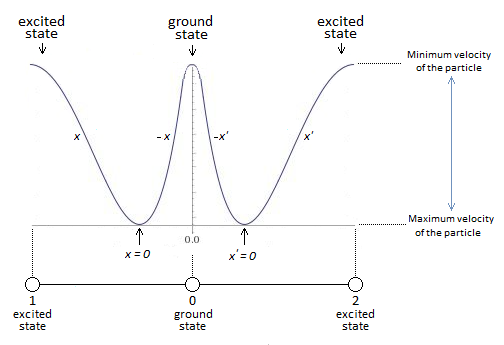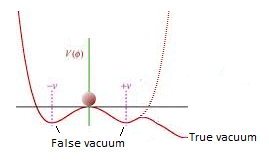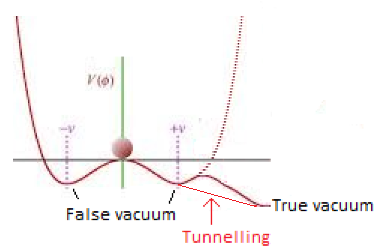# Anharmonic Oscillator

## Recommended Posts

The gauge groups I mentioned are not specifically the harmonic oscillator. QFT accounts for the quantum harmonic oscillator in its gauge groups but that isn't the full story.

Here is a primer QFT takes a considerable time to learn.

In order to be observed there must be a quanta of action. The external lines on those Feymann diagrams are observable's. (Operators) The internal lines (propogators ) (often thought of as virtual particles) are not observable.

Here is the standard model of particle physics (not including Higgs) that is in another post

$\mathcal{G}=SU(3)_c\otimes SU(2)_L\otimes U(1)_Y$

Color, weak isospin, abelion Hypercharge groups.

Couplings in sequence $g_s, g, \acute{g}$

$\mathcal{L}_{gauge}=-\frac{1}{2}Tr{G^{\mu\nu}G_{\mu\nu}}-\frac{1}{2}Tr {W^{\mu\nu}W_{\mu\nu}}-\frac{1}{4}B^{\mu\nu}B_{\mu\nu}$

Field strengths in sequence in last G W B tensors for SU(3),SU(2) and U(1)

$D_\mu=\partial_\mu+ig_s\frac{\lambda_i}{2}G^i_\mu+ig\frac{\sigma_i}{2}W^i_\mu+igQ_YB_\mu$

Corresponds to

$G_{\mu\nu}=-\frac{i}{g_s}[D_\mu,D_\nu]$

$W_-\frac{I}{g}[D_{\mu}D_{\nu}]$

$B_{\mu\nu}-\frac{I}{\acute{g}}[D_\mu,D_\nu]$

You can find the Higgs on page two

In essence we were trying to validate and reverse engineer the equation in post 1. In order to do that I had to examine each gauge group of the standard model.

Given by

$\mathcal{G}=SU(3)_c\otimes SU(2)_L\otimes U(1)_Y$

Edited by Mordred
• Replies 276
• Created

#### Popular Posts

The gauge groups I mentioned are not specifically the harmonic oscillator. QFT accounts for the quantum harmonic oscillator in its gauge groups but that isn't the full story.  https://www.google.

Sigh no you have to recognize that harmonic, inharmonic and anharmonic are descriptives of the characteristics of the oscillator.  Here is how classical harmonic oscillator is described.  A

Be careful of such bold statements for example try to define that statement above to the Higgs coupling constants. First ask yourself the function of a coupling constant in regards to mass. (Hint

#### Posted Images

32 minutes ago, Mordred said:

Here is the standard model of particle physics (not including Higgs) that is in another post

G=SU(3)cSU(2)LU(1)Y

Color, weak isospin, abelion Hypercharge groups.

Couplings in sequence gs,g,g´

Lgauge=12TrGμνGμν12TrWμνWμν14BμνBμν

Field strengths in sequence in last G W B tensors for SU(3),SU(2) and U(1)

This makes the link between the strong interaction, the weak interaction, and quantum electrodynamics, right? Do we use the same coupling constant? Is it proportional?

Edited by Kartazion
LaTeX
##### Share on other sites

There is 18 coupling constants in total for the weak,  strong and Electromagnetic force including the two Higgs coupling constants but yes we examine all four of the above in that thread.

GR gauge group would best be described as the Poincare group SO (3.1)

Last I recall supersymmetry had roughly 114 coupling constants lol.

Edited by Mordred
##### Share on other sites

The coupling constant can be measured with the potential energy well of the harmonic oscillator. In fact, the interaction becomes weaker when the particle converges to its ground state.
In other words the levels of the coupling force is proportional to x.

Edited by Kartazion
##### Share on other sites

Be careful of such bold statements for example try to define that statement above to the Higgs coupling constants.

First ask yourself the function of a coupling constant in regards to mass. (Hint).

Mass is resistance to inertia change. (Second hint)

You might also want to better understand Langrangian and the principle of least action with the above.

QFT is based on perturbation theory which employs action in accordance to that principle.

Here this will help call it the QFT preliminary Ie chapter 1.

QFT can be described as a coupling of SR and QM in the non relativistic regime.

1) Field :A field is a collection of values assigned to geometric coordinates. Those values can be of any nature and does not count as a substance or medium.

2) As we are dealing with QM we need the simple quantum harmonic oscillator

3) Particle: A field excitation

Simple Harmonic Oscillator

$\hat{H}=\hbar w(\hat{a}^\dagger\hat{a}+\frac{1}{2})$

the $\hat{a}^\dagger$ is the creation operator with $\hat{a}$ being the destruction operator. $\hat{H}$ is the Hamiltonian operator. The hat accent over each symbol identifies an operator. This formula is of key note as it is applicable to particle creation and annihilation. $\hbar$ is the Planck constant (also referred to as a quanta of action) more detail later.

Heisenberg Uncertainty principle

$\Delta\hat{x}\Delta\hat{p}\ge\frac{\hbar}{2}$

$\hat{x}$ is the position operator, $\hat{p}$ is the momentum operator. Their is also uncertainty between energy and time given by

$\Delta E\Delta t\ge\frac{\hbar}{2}$ please note in the non relativistic regime time is a parameter not an operator.

Physical observable's are operators. in order to be a physical observable you require a minima of a quanta of action defined by

$E=\hbar w$

Another key detail from QM is the commutation relations

$[\hat{x}\hat{p}]=\hat{x}\hat{p}-\hat{p}\hat{x}=i\hbar$

Now in QM we are taught that the symbols $\varphi,\psi$ are wave-functions however in QFT we use these symbols to denote fields. Fields can create and destroy particles. As such we effectively upgrade these fields to the status of operators. Which must satisfy the commutation relations

$[\hat{x}\hat{p}]\rightarrow[\hat{\psi}(x,t),\hat{\pi}(y,t)]=i\hbar\delta(x-y)$

$\hat{\pi}(y,t)$ is another type of field that plays the role of momentum

where x and y are two points in space. The above introduces the notion of causality. If two fields are spatially separated they cannot affect one another.

Now with fields promoted to operators one wiill wonder what happen to the normal operators of QM. In QM position $\hat{x}$ is an operator with time as a parameter. However in QFT we demote position to a parameter. Momentum remains an operator.

In QFT we often use lessons from classical mechanics to deal with fields in particular the Langrangian

$L=T-V$

The Langrangian is important as it leaves the symmetries such as rotation invariant (same for all observers). The classical path taken by a particle is one that minimizes the action

$S=\int Ldt$

the range of a force is dictated by the mass of the guage boson (force mediator)

$\Delta E=mc^2$ along with the uncertainty principle to determine how long the particle can exist

$\Delta t=\frac{\hbar}{\Delta E}=\frac{\hbar}{m_oc^2}$ please note we are using the rest mass (invariant mass) with c being the speed limit

$velocity=\frac{distance}{time}\Rightarrow\Delta{x}=c\Delta t=\frac{c\hbar}{mc^2}=\frac{\hbar}{mc^2}$

from this relation one can see that if the invariant mass (rest mass) m=0 the range of the particle is infinite. Prime example gauge photons for the electromagnetic force.

Lets return to $L=T-V$ where T is the kinetic energy of the particle moving though a potential V using just one dimension x. In the Euler-Langrange we get the following

$\frac{d}{dt}\frac{\partial L}{\partial\dot{x}}-\frac{\partial L}{\partial x}=0$ the dot is differentiating time.

Consider a particle of mass m with kinetic energy $T=\frac{1}{2}m\dot{x}^2$ traveling in one dimension x through potential $V(x)$

Step 1) Begin by writing down the Langrangian

$L=\frac{1}{2}m\dot{x}^2-V{x}$

next is a derivative of L with respect to $\dot{x}$ we treat this as an independent variable for example $\frac{\partial}{\partial\dot{x}}(\dot{x})^2=2\dot{x}$ and $\frac{\partial}{\partial\dot{x}}V{x}=0$ applying this we get

step 2)

$\frac{\partial L}{\partial\dot{x}}=\frac{\partial}{\partial\dot{x}}[\frac{1}{2}m\dot{x}^2]=m\dot{x}$

which is just mass times velocity. (momentum term)

step 3) derive the time derivative of this momentum term.

$\frac{d}{dt}\frac{\partial L}{\partial\dot{x}}=\frac{d}{dt}m\dot{x}=\dot{m}\dot{x}+m\ddot{x}=m\ddot{x}$ we have mass times acceleration

Step 4) Now differentiate L with respect to x

$\frac{\partial L}{\partial x}[\frac{1}{2}m\dot{x}^2]-V(x)=-\frac{\partial V}{\partial x}$

Step 5) write the equation to describe the dynamical behavior of our system.

$\frac{d}{dt}(\frac{\partial L}{\partial\dot{x}}-\frac{\partial L}{\partial x}=0$$\Rightarrow\frac{d}{dt}$$(\frac{\partial L}{\partial\dot{x}})$$=\frac{\partial L}{\partial x}\Rightarrow m\ddot{x}=-\frac{\partial V}{\partial x}$

recall from classical physics $F=-\nabla V$ in 1 dimension this becomes $F=-\frac{\partial V}{\partial x}$ therefore $\frac{\partial L}{\partial x}=-\frac{\partial V}{\partial x}=F$ we have $m\ddot{x}-\frac{\partial V}{\partial x}=F$

Edited by Mordred
##### Share on other sites

The Higgs Mexican hat potential does not below it's vacuum expectation value.

Not all coupling constants obey the same relations to other coupling constants they each have their own potential curves. In essence any equation that describes your potential graph will not match the one I posted.

Edited by Mordred
##### Share on other sites

I found these two explanations:

Back to previous graph:##### Share on other sites
12 hours ago, Mordred said:

Last I recall supersymmetry had roughly 114 coupling constants lol.

It's 114 different kinds? Or so that means that the coupling constant does not follow a function?

On 12/27/2019 at 5:11 AM, Mordred said:

The Higgs Mexican hat potential does not below it's vacuum expectation value.

Does this mean that the ground state cannot be in the true vacuum?

A potential with two false vacua, φ = φ A , φ B , and one true vacuum, φ = φ C
Where φA is Higgs' false vacuum. But how to incorporate the true vacuum into the Higgs field?

##### Share on other sites

The Higgs vacuum expectation value is 246 Gev.  It is always a non zero value. One has to be careful on how one defines a true vacuum. For example a true vacuum could mean the Einstein Vacuum which is devoid of all particles. However with zero point energy there is always quantum fluctuations leading to particle production.

7 hours ago, Kartazion said:

It's 114 different kinds? Or so that means that the coupling constant does not follow a function

Every coupling constant is defined by its own function. This is true for the SM coupling constants as well as those in Supersymmetry models.

##### Share on other sites
40 minutes ago, Mordred said:

Every coupling constant is defined by its own function. This is true for the SM coupling constants as well as those in Supersymmetry models.

I only found that of the four forces. Where to find others?1 hour ago, Mordred said:

The Higgs vacuum expectation value is 246 Gev.  It is always a non zero value. One has to be careful on how one defines a true vacuum. For example a true vacuum could mean the Einstein Vacuum which is devoid of all particles. However with zero point energy there is always quantum fluctuations leading to particle production.

Can I define the true vacuum as the graph below if The Higgs vacuum expectation value is 246 Gev?It is certain that if the system were at zero fluctuation of the particle at zero point energy, then we could not exist.

##### Share on other sites

Though you would need to go through the CKMS and PMNS mixing angles via the unitary triangle mentioned in the above article for the mass mixing angles for each particle species in each generation.

False vacuum is a higher state than the true vacuum. You will also need to ensure their is a potential barrier between the two for quantum tunnelling from the false vacuum to the true vacuum.

Edited by Mordred
##### Share on other sites
29 minutes ago, Mordred said:

False vacuum is a higher state than the true vacuum. You will also need to ensure their is a potential barrier between the two for quantum tunnelling from the false vacuum to the true vacuum.Edited by Kartazion
##### Share on other sites

No you have one too many humps the false and true  vacuum will have their own minima with the minima of the false vacuum higher than the true vacuum. The tunneling must occur from the  false vacuum to true vacuum. Through the potential barrier between them.

Here is an older paper of false vacuum. It was widely in inflationary cosmology. Allen Guth first applied it to inflation however Coleman was also using false vacuum at roughly the same time period.

This is an older application however it points out all the essential details.

##### Share on other sites
4 hours ago, Mordred said:

No you have one too many humps the false and true  vacuum will have their own minima with the minima of the false vacuum higher than the true vacuum. The tunneling must occur from the  false vacuum to true vacuum. Through the potential barrier between them.

Theory before the Higgs find.
Source: Will the LHC Look into the Fate of the Universe? http://inspirehep.net/record/790889##### Share on other sites

Hello.

I see that this paper is relevant in the context of the imaginary part of the ground state of the harmonic oscillator in relation to the coupling constant.

##### Share on other sites

On 12/26/2019 at 3:08 PM, Kartazion said:

… I deduce that our universe works with a single moving particle.

On 12/26/2019 at 4:53 PM, Mordred said:

... In essence symmetry gauge theory covers all the aspects of the above without specifying a single particle at all spacetime locations.

Now under QFT the particle number density varies accordingly to the field strength.

##### Share on other sites

That's definitely a view I share, in my 36 years of studying particle physics including my degree. One learns that a particle is not some corpuscular solid. The pointlike attributes can be described by the De Broglie and Compton wavelength.

Good article above its one Of commonly refer to, if you focus your learning upon the above understanding you will get a solid grasp.

I have often stated "solid is an illusion".

Funny part was when I first started learning particle physics I was a strong particle vs waveform advocate.

Edited by Mordred
##### Share on other sites

I deduced from this that matter only appears when we look at it. The rest is only a wave of probability of presence of the particle. The quantum system is suddenly lazy.

On 12/27/2019 at 2:11 AM, Kartazion said:

It's why Albert Einstein said: "I like to think the Moon is there even if I am not looking at it."

##### Share on other sites

Just be careful there is a difference between wave probability functions and physical wave functions.  The probability functions gives the likely hood of finding the property associated with a given particle at a location.

Edited by Mordred
##### Share on other sites
• 2 weeks later...

But we can see some correspondence between a wave function and the probability distribution for one particle in quantum mechanics, no?

I found that:

Definition (one spinless particle in one dimension) Position-space wave functions
If the particle's position is measured, its location cannot be determined from the wave function, but is described by a probability distribution.

###################################

I would like to know if there is a link between the harmonic oscillator, and the time translation symmetry?##### Share on other sites

It's still weird as a novice to get there ... So how is it that starting from a simple oscillator I arrive at the foundations of quantum mechanics?
I never said it works with a single particle for fun. It is a practicality deduction.

Always and with the an·harmonic oscillator I will post something in relativistic physics related to time dilation.

##### Share on other sites

All particles can be described via wavefunctions the Schrodinger equations can be time dependent and time independent so time symmetry relations would certainly be involved. CPT involves time symmetry.

##### Share on other sites

If you want to learn quantum mechanics you have to understand the an·harmonic oscillator.
We can say that our universe is then an an·harmonic oscillator.
It is the future.

## Create an account

Register a new account